## Example Questions

### Example Question #11 : Percentage

A politician promises to spend a minimum of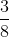of his budget on improving roads. What is the minimum percent of the budget that must be spent on roads to keep this promise?

Possible Answers: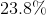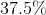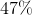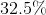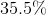Correct answer:Explanation:

To find a percentage from a fraction, multiply the numerator by, then solve the fraction.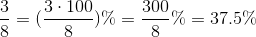Thus, the politician must spend at leastof the budget on roads.

### Example Question #11 : Percentage

Convert to a percentage: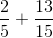Round to the nearest integer.

Possible Answers: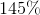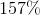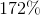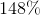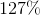Correct answer:Explanation:

We can solve this problem either by adding the two fractions and then converting to a percentage, or by converting each fraction to a percentage before adding them. We'll use the first method in this case: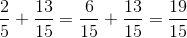Now, just as before, multiply our numerator byand solve the fraction: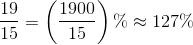### Example Question #11 : Percentage

The girl's tennis team decided to do multiple fundraisers to raise enough to buy new equipment for next season. The following is a table of 4 of the fundraisers they did and the fraction of the total funds each raised.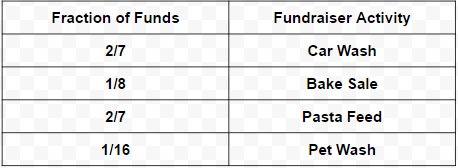The last fundraiser they did earned them the final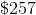they needed. What percent of the total funds raised did the last fundraiser raise?

Possible Answers: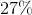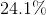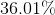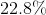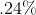Correct answer:Explanation:

The question asks to find what percent the last fundraiser makes up of the total funds raised. The information that's given is how much that last fundraiser made and what fraction the other fundraisers made.

This problem may be solved without the amount of money the last fundraiser raised. The reason is that we know that only 5 fundraisers were done. We're given what the fraction of the other fundraisers were.

If we were to add all the fractions together, including the last fundraiser, the sum of all would equal 1.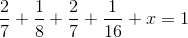Fractions are another version of decimals but also another version of percentages. Percentages are essentially a prettier way of showing parts (or fractions) of a whole, which would be 100%. In the case of fractions, this is the same logic, which is why when all the fractions are summed, they equal 1. This 1 represents 100%.
Upon using lowest common denominators, adding the fractions together, and solving for x, x is calculated to be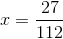We've almost obtained the final answer. Now it's just a matter of converting this fraction into a percent. As mentioned previously, fractions are another version of decimals. Think of decimals as being the middle conversion between fractions and percents. All that needs to be done to a decimal to turn it in to a percent is to multiply it by 100. For instance, .50 becomes 50%. Conversely, .50 is the same asin fraction form. Therefore, one half is 50%.
In order to solve for x in percent, the same conversion must be done.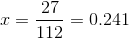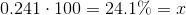### Example Question #11 : Percentage

Convert the following to a percentage.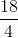Possible Answers: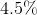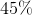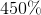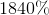Correct answer:Explanation:

To convert a fraction into a percentage, simply simplify the fraction into a decimal and multiply by 100.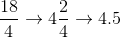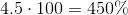### Example Question #12 : Percentage

If a dress in a store originally costs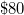, what does it cost (in dollars) after a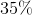discount is applied?

Possible Answers:

$63$52

$70$28

$45 Correct answer:$52

Explanation:

To findof 80,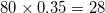. Next, subtract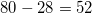, for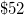as the final answer.

### Example Question #1 : How To Find The Part From The Whole With Percentage

If n% of 400 is 260, then what is 20% of n?

Possible Answers:

15

31

25

14

13

Correct answer:

13

Explanation:

n% of 400 is 260

A number as a percentage of a second number is equal to the first number divided by the second number, times 100%.

260 / 400 * 100% = .65 * 100% = 65%. n = 65.

20% of n?

20% of 65 is equal to 65 * 20 / 100 = 65 / 5 = 13.

### Example Question #11 : Percentage

Auditions are being held for the annual school musical.  There are 18 roles available in the show.  If 75 students try out, what percentage of the students will get a role in the musical?

Possible Answers:

26%

22%

30%

28%

24%

Correct answer:

24%

Explanation:

Percentage is #roles / # students = 18 / 75 = 0.24 = 24%

### Example Question #1 : How To Find The Part From The Whole With Percentage

You are making cookies and mixing the dry ingredients: flour, white sugar and brown sugar. If the recipe calls for 3 1/4 cups of flour, 1/2 cup of white sugar and 3/4 cup of brown sugar, what percentage of the recipe is white sugar?

Possible Answers:

16.7%

11.1%

17.5%

20%

10%

Correct answer:

11.1%

Explanation:

Total ingredients = 3 1/4 cups + 1/2 cup + 3/4 cup = 4 1/2 cups

White sugar percentage = white sugar / total = (1/2)/(9/2) = .111 = 11.1%

### Example Question #1 : How To Find The Part From The Whole With Percentage

Jamie bought shoes for 30% off the regular price of \$37.99, rounded to the nearest cent. If she wanted to use exact change to pay, how much would she need in coins?

Possible Answers: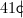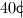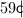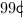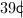Correct answer:Explanation: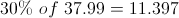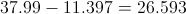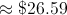### Example Question #1 : How To Find The Part From The Whole With Percentage

A class has 25 students. If 60% of them are boys, how many students are girls?

Possible Answers:

10

12

15

18

Correct answer:

10

Explanation:

If 60% of the students are boys, 40% are girls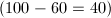. Multiply 25 by 40%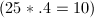. Therefore, 10 students are girls.

### All ACT Math Resources